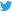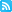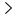# ferris blogs stuff

Technical ramblings and other stuffTwitterRSS

ferris blogs stuffBogus paper pseudocode: A pyramid vector quantizer

30 May 2021

## Bogus paper pseudocode: A pyramid vector quantizer

When implementing an algorithm from a paper/blog post/whatever, it’s not uncommon to transcribe the included (pseudo)code while trying to understand the ideas presented. Unfortunately, this code is often presented in a poor manner, and in some cases, is just plain wrong. This is particularly annoying when the paper is behind a paywall. I’ve encountered this enough times that I think I should start writing quick posts explaining my findings and how to fix them. This is the first of those posts.

The decoder pseudocode in T. Fischer’s “A pyramid vector quantizer” is awful. It’s presented in a near-unreadable fashion that’s difficult to transcribe into basically any programming language, and what’s worse, it’s missing a crucial detail in step 3 that doesn’t adjust `xb` to account for the decoded sign for dimension `i`, that causes the decoder to de-sync for the remaining dimension(s). The simplest pathological case I found was `b = 4, L = 2, K = 2`.

Actually, a really funny thing is that if you transcribe the code directly, it will work, so long as your environment allows integer overflows and you don’t mind waiting a while. But of course, this is not usable or helpful.

The encoder is not much better; the 3 cases in step 1 can be collapsed to just two (the conditional is simply “is `x``i` nonzero”); the case with a single pulse vs the case with multiple pulses are unhelpful to distinguish in this way (just naming the terms would have been a better approach).

Anyways, there’s not much more to say about it, but here’s some (working!) rust code that demonstrates pulse vector encoding and decoding with notation/conventions that reflect the paper, so you can learn from this instead. I’ve also split the encoder’s `b` adjustment into a few terms and tried to match those terms in the decoder. It should be much easier to follow this way (as it was much easier to debug!):

``````fn l1_norm(pulse_vector: &[i32]) -> u32 {
pulse_vector.iter().map(|&x| x.abs() as u32).sum()
}

#[allow(non_snake_case)]
fn N(L: u32, K: u32) -> u32 {
debug_assert!(L >= 0);
debug_assert!(K >= 0);
if K == 0 {
return 1;
}
if L == 0 {
return 0;
}
N(L - 1, K) + N(L - 1, K - 1) + N(L, K - 1)
}

#[allow(non_snake_case)]
fn pvq_encode(x: &[i32]) -> u32 {
let L = x.len() as u32;
let K = l1_norm(x);
let mut b = 0;
let mut i = 1;
let mut k = K;
let mut l = L;
while k != 0 {
let x_i = x[(i - 1) as usize];
if x_i != 0 {
let j = x_i.abs() as u32;
let non_zero_offset = N(l - 1, k);
let additional_pulse_offsets = 2 * (1..j).map(|j| N(l - 1, k - j)).sum::<u32>();
let sign_offset = ((1 - x_i.signum()) / 2) as u32 * N(l - 1, k - j);
b += non_zero_offset
+ additional_pulse_offsets
+ sign_offset;
k -= j;
}
l -= 1;
i += 1;
}
b
}

#[allow(non_snake_case)]
fn pvq_decode(b: u32, L: u32, K: u32) -> Vec<i32> {
let mut x = vec![0; L as usize];
let mut xb = 0; // This corresponds to b in the encoder
let mut i = 1;
let mut k = K;
let mut l = L;
while k != 0 {
let non_zero_offset = N(l - 1, k);
if b >= xb + non_zero_offset {
xb += non_zero_offset;
let mut j = 1;
// additional_pulse_offsets
loop {
let additional_pulse_offset = 2 * N(l - 1, k - j);
if b < xb + additional_pulse_offset {
break;
}
xb += additional_pulse_offset;
j += 1;
}
let sign_offset = N(l - 1, k - j);
x[(i - 1) as usize] = if b >= xb + sign_offset {
// Note: This is the crucial adjustment that's just plain missing from the paper!
//  Without this, xb is no longer in sync with b in the encoder after this dimension
xb += sign_offset;
-(j as i32)
} else {
j as i32
};
k -= j;
}
l -= 1;
i += 1;
}
x
}
``````

Now, I’m well aware that the naming convention here does not match that which you’d find in the wikipedia article or other sources; this is also annoying, but the mapping isn’t too bad once you have working code to begin with, and since I was trying to understand the original paper, I wanted to use the notation that was used there. Oddly enough this improvement paper also uses the original notation again. I’m also well aware that this is not the most efficient embodiment of this algorithm by any means, but again, I was trying to understand the original paper.

Last thing, here’s the test driver I tested the code with:

``````struct Lcg {
state: u32,
}

impl Lcg {
fn new(state: u32) -> Lcg {
Lcg {
state,
}
}

fn next_u32(&mut self) -> u32 {
let ret = self.state;
// Numerical Recipes parameters
self.state = self.state.wrapping_mul(1664525).wrapping_add(1013904223);
ret
}
}

fn test_pulse_vector(pulse_vector: &[i32]) {
println!("vector: {:?}", pulse_vector);
let L = pulse_vector.len() as u32;
let K = l1_norm(pulse_vector);
println!(" - L = {}, K = {}, N(L, K) = {} ({} bits)", L, K, N(L, K), (N(L, K) as f64).log2());
let codeword = pvq_encode(&pulse_vector);
println!(" - codeword: {}", codeword);
let decoded_pulse_vector = pvq_decode(codeword, L, K);
println!(" - decoded vector: {:?}", decoded_pulse_vector);
assert_eq!(decoded_pulse_vector, pulse_vector);
}

fn main() {
let pulse_vector = vec!;
test_pulse_vector(&pulse_vector);
let pulse_vector = vec![-1];
test_pulse_vector(&pulse_vector);

let pulse_vector = vec![1, -1];
test_pulse_vector(&pulse_vector);

let pulse_vector = vec![0, 1, 0, -1];
test_pulse_vector(&pulse_vector);

let L = 20;
let K = 10;
let mut lcg = Lcg::new(0);
let mut pulse_vector = vec![0i32; L];
for _ in 0..K {
let i = lcg.next_u32() as usize % L;
let sign = if pulse_vector[i] == 0 {
(lcg.next_u32() & 1) as i32 * 2 - 1
} else {
pulse_vector[i].signum()
};
pulse_vector[i] += sign;
}
test_pulse_vector(&pulse_vector);

for i in 0..4 {
let L = 2;
let K = 1;
let pulse_vector = pvq_decode(i, L, K);
let codeword = pvq_encode(&pulse_vector);
println!("{} -> {:?} -> {}", i, pulse_vector, codeword);
assert_eq!(i, codeword);
}

for i in 0..8 {
let L = 2;
let K = 2;
let pulse_vector = pvq_decode(i, L, K);
let codeword = pvq_encode(&pulse_vector);
println!("{} -> {:?} -> {}", i, pulse_vector, codeword);
assert_eq!(i, codeword);
}
}
``````
``````vector: 
- L = 1, K = 1, N(L, K) = 2 (1 bits)
- codeword: 0
- decoded vector: 
vector: [-1]
- L = 1, K = 1, N(L, K) = 2 (1 bits)
- codeword: 1
- decoded vector: [-1]
vector: [1, -1]
- L = 2, K = 2, N(L, K) = 8 (3 bits)
- codeword: 3
- decoded vector: [1, -1]
vector: [0, 1, 0, -1]
- L = 4, K = 2, N(L, K) = 32 (5 bits)
- codeword: 9
- decoded vector: [0, 1, 0, -1]
vector: [1, 0, 2, -2, 1, -1, 0, -1, 0, -1, 0, 0, 0, 0, 0, 0, 0, -1, 0, 0]
- L = 20, K = 10, N(L, K) = 3339504032 (31.636986710067298 bits)
- codeword: 2330460419
- decoded vector: [1, 0, 2, -2, 1, -1, 0, -1, 0, -1, 0, 0, 0, 0, 0, 0, 0, -1, 0, 0]
0 -> [0, 1] -> 0
1 -> [0, -1] -> 1
2 -> [1, 0] -> 2
3 -> [-1, 0] -> 3
0 -> [0, 2] -> 0
1 -> [0, -2] -> 1
2 -> [1, 1] -> 2
3 -> [1, -1] -> 3
4 -> [-1, 1] -> 4
5 -> [-1, -1] -> 5
6 -> [2, 0] -> 6
7 -> [-2, 0] -> 7
``````

30 May 2021